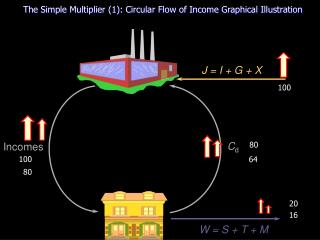DownloadDownload PresentationC d

# C d

Download Presentation## C d

- - - - - - - - - - - - - - - - - - - - - - - - - - - E N D - - - - - - - - - - - - - - - - - - - - - - - - - - -
##### Presentation Transcript

1. 100 80 100 64 80 20 16 The Simple Multiplier (1): Circular Flow of Income Graphical Illustration J = I + G + X Cd Incomes fig W = S + T + M

2. a Ye1 The simple multiplier (2): Shift in Injections Graphical Illustration W, J W J1 O fig Y

3. b Ye2 The simple multiplier (2): Shift in Injections Graphical Illustration W, J W J2 a J1 O fig Ye1 Y

4. Multiplier = DY / D J = DY / DW = c-a / b-c DW DJ c DY The simple multiplier (2): Shift in Injections Graphical Illustration W, J W b J2 J2 a J1 J1 O fig Ye1 Ye2 Y

5. The simple multiplier (3): Shift in Withdrawals Graphical Illustration W, J W1 a J O fig Ye1 Y

6. c Ye2 The simple multiplier (3): Shift in Withdrawals Graphical Illustration W, J W1 W2 a J O fig Ye1 Y

7. Multiplier = DY / DW = c-a / a-b b DW DY The simple multiplier (3): Shift in Withdrawals Graphical Illustration W, J W1 W2 a c J O Ye1 Y Ye2 fig

8. Ye1 The simple multiplier (4): Shift in AE Graphical Illustration E, W, J Y E1 O Y fig

9. The simple multiplier (4): Shift in AE Graphical Illustration E, W, J Y E2 E1 O Ye1 Ye2 Y fig

10. Multiplier = DY / DJ = c-a / b-a c DY b DJ The simple multiplier (4): Shift in AE Graphical Illustration E, W, J Y E2 E1 a O Ye1 Ye2 fig Y